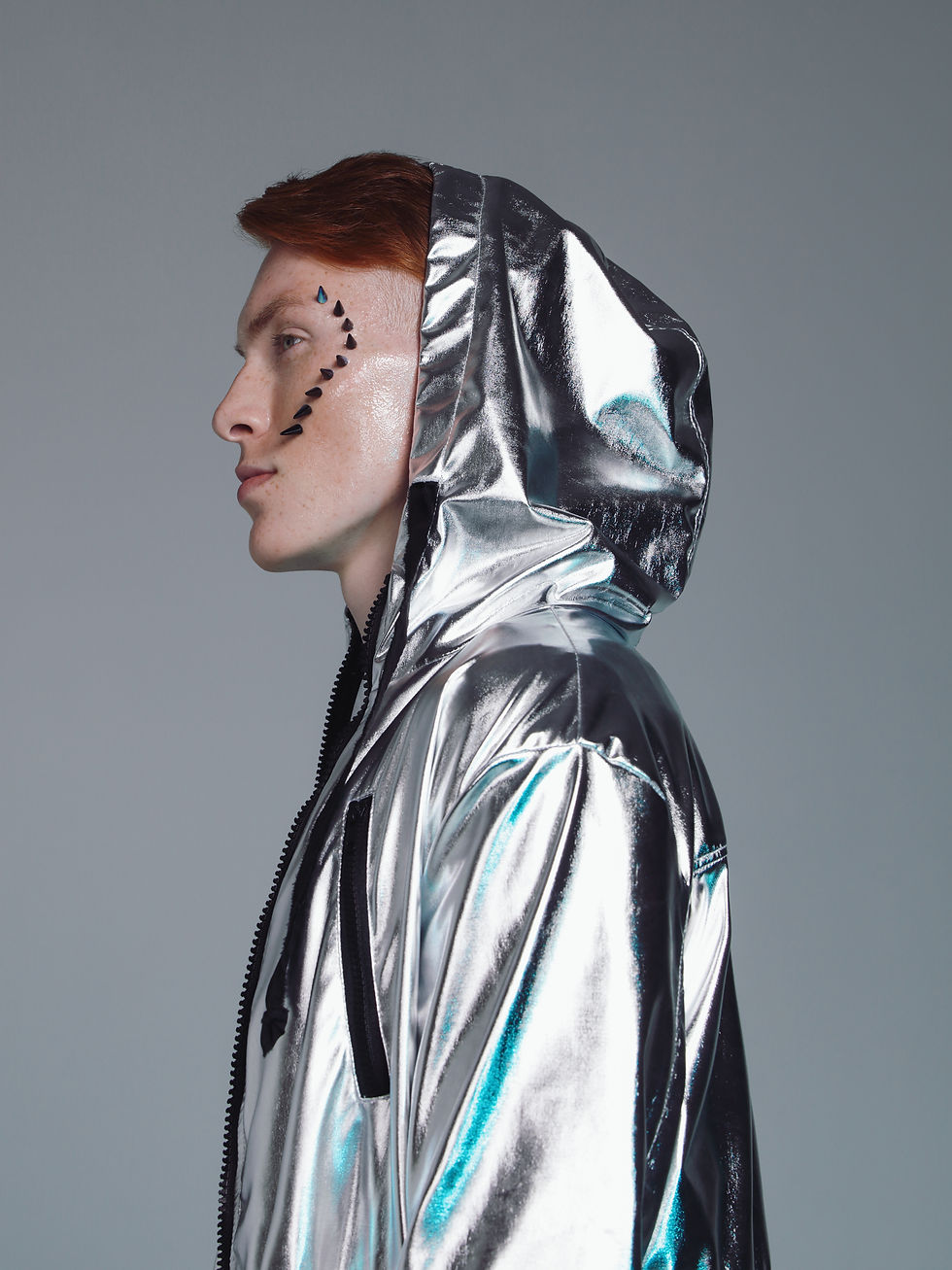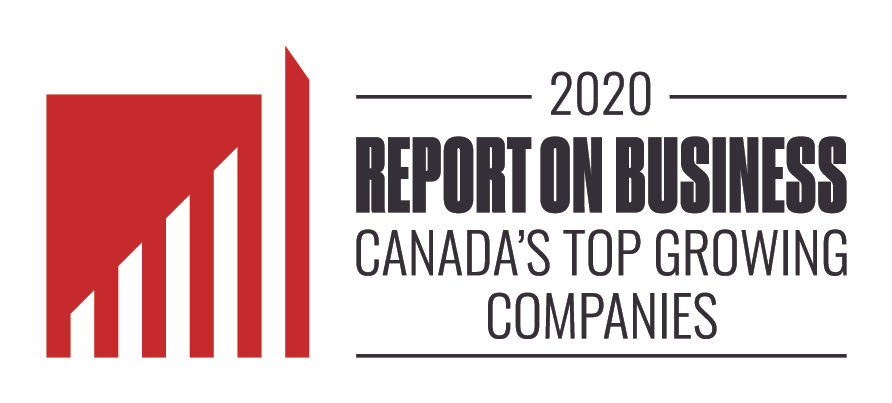Spectrums### PARHumans “see” Lumens – Plants “see” PAR The type of light that is ‘visible’ and usable to a plant is far different than what the human eye can see. The type of light that a plant can ‘see’ is far greater. How grow lights efficacy and light output is measured is different than traditional light systems. Plant spectrum is often referred to as PAR (Photosynthetically Active Radiation).

PAR = The type of light that drives the majority of photosynthesis. The visible light from 400-700nm. Measured in total energy(watts/meter2)

New studies are revealing that some plants effectively use light beyond the traditional PAR range. Plants have shown effective growth and reaction between 380 and 780nm. We call this the Plant Spectrum or ePAR (Extended PAR).As PAR is the type of light that is to be measured. The method of measurement for grow lights is different than regular lights. This number is expressed as PPF (Photosynthetic Photon Flux) or the measurement of the total photons of PAR that is emitted from the light fixture. This is expressed as µmol/s (micromoles per second). This number is the key to measure the overall quality of a grow light. This number helps to compare the efficacy of one grow light to another.

This only measures the light from 400nm-700nm, as these are the wavelengths that most contribute to photosynthesis.

PPF cannot measure the amount of light delivered to the plant, nor the direction of the light emitted from the fixture. PPF can only be done in laboratory setting, in a calibrated integration sphere.

### PPFAll grow lights are measured in moles (mol), or micromoles (µmol) (one-millionth of a mole). This is a measurement of the number of photons emitted over a period of type on a specific surface.

Measurement of µmol is required as grow lights need to measure the type of light, as well as the intensity of the light. In the context of grow lights this is expressed as µmol/m2/s or micromoles per meter square per second. This is measuring the quantity of the light (in photons) from second to second.

PPFD – Photosynthetic Photon Flux Density – how many µmol are delivered. Typically measured in 1 square meter.

This is the performance of a light fixture output and is the amount of light that a plant requires for adequate or successful growth

### PPFDLast but not least, PPE (Photosynthetic Photon Efficacy). The measurement of the efficiency of the fixture to convert energy (watts) into light (PPF). This measurement is calculated by the µmol/s divided by the input power (watts). One watt equals one joule per second (J/s). The measurement is expressed as µmol/J That all sounds complicated. However, this is an effective method to compare one type of grow light to another. However, it should not be the sole factor of consideration.

All factors should be considered when comparing one light to another. This includes PAR, PPF, PPFD and PPE. This method can be used to compare different types of light types to each other, including LED, fluorescent, HPS and induction.

### PPEGrow lights can have a hefty price tag. Controlling all aspects of the light output to reduce the amount of energy required can have a significant effect on the bottom line of your operations.

The cost that should be considered is the cost per micromole – not just the cost per fixture, or the cost per watt. The cost per µmol/J – is also a method to compare light types to each other.

### COST PER µmol/JThe chart indicates that the initial costs per µmol for Fixture 2 is better. However, this is not the whole picture of costs, consider lifetime factors, and costs per watt to operate the fixture as well.# Common Terms ExplainedDaily Light Integral is the measurement of the PPFD delivered to an area of 1-meter squared (1M2) over a 24-hour period.  Measured within the standard PAR (400-700nm) range only.

Eg. a Mature Tomato plant requires between 22-30 DLI. To calculate the required brightness and time of the light to be “on” would be:

600 PPFD x (3,600 x12) / 1,000,000 = 25.9 DLI

Thus the target would be 600 PPFD over a 12 hour period.

### DLIConnect With Us

LED Smart Inc.

18905 32nd Avenue

Surrey, BC V3Z 1A7

1.800.457.0416

info@ledsmart.com

sales@ledsmart.com